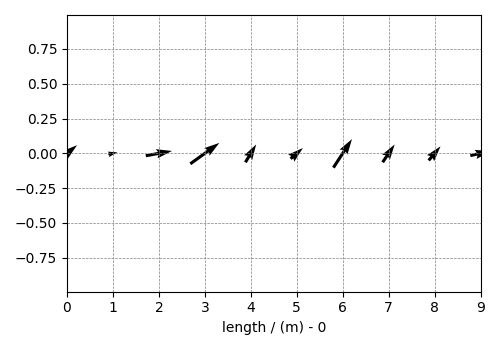# Vector, 1D{2} dataset¶

The 1D{2} datasets are one-dimensional, $$d=1$$, with two-component dependent variable, $$p=2$$. Such datasets are more common with the weather forecast, such as the wind velocity predicting at a location as a function of time.

The following is an example of a simulated 1D vector field dataset.

import matplotlib.pyplot as plt

import csdmpy as cp

filename = "https://www.ssnmr.org/sites/default/files/CSDM/vector/1D_vector.csdf"
print(vector_data.data_structure)

{
"csdm": {
"version": "1.0",
"timestamp": "2019-02-12T10:00:00Z",
"dimensions": [
{
"type": "linear",
"count": 10,
"increment": "1.0 m",
"quantity_name": "length",
"reciprocal": {
"quantity_name": "wavenumber"
}
}
],
"dependent_variables": [
{
"type": "internal",
"numeric_type": "float32",
"quantity_type": "vector_2",
"components": [
[
"0.6907923, 0.31292602, ..., 0.40570852, 0.7005596"
],
[
"0.5603441, 0.06866818, ..., 0.48200375, 0.15077808"
]
]
}
]
}
}


The tuple of the dimension and dependent variable instances from this example are

x = vector_data.dimensions
y = vector_data.dependent_variables


with coordinates

print(x.coordinates)

[0. 1. 2. 3. 4. 5. 6. 7. 8. 9.] m


In this example, the components of the dependent variable are vectors as seen from the quantity_type attribute of the corresponding dependent variable instance.

print(y.quantity_type)

vector_2


From the value vector_2, vector indicates a vector dataset, while 2 indicates the number of vector components.

Visualizing the dataset

plt.figure(figsize=(5, 3.5))
cp.plot(vector_data)
plt.tight_layout()
plt.show()Total running time of the script: ( 0 minutes 0.247 seconds)

Gallery generated by Sphinx-Gallery